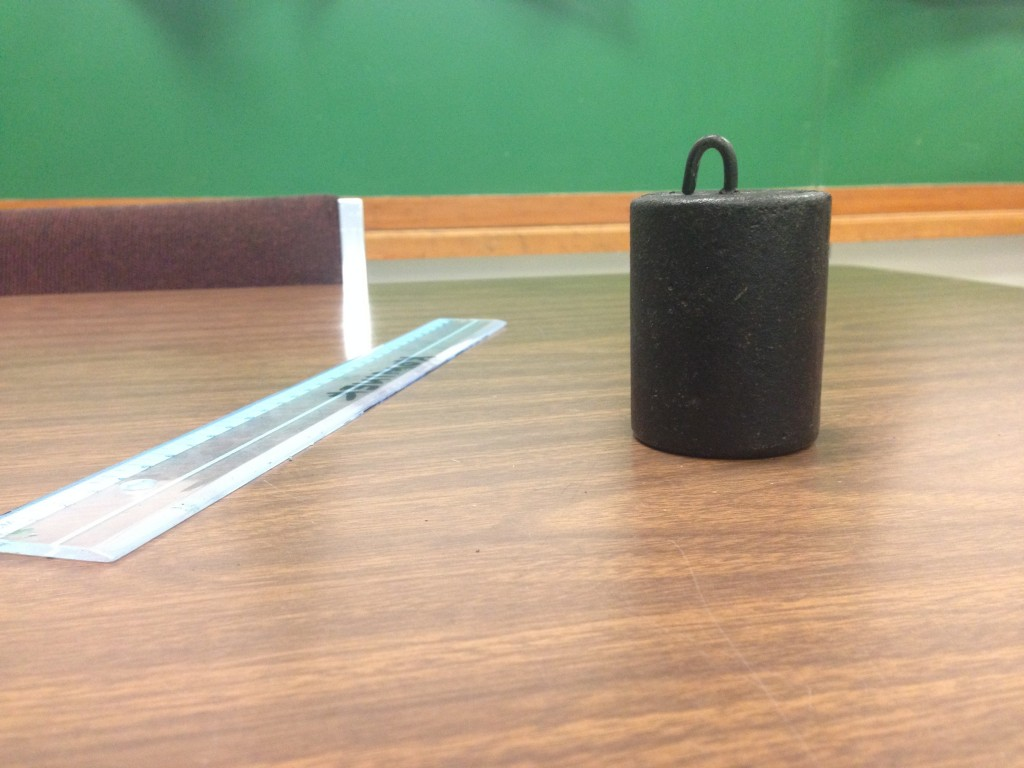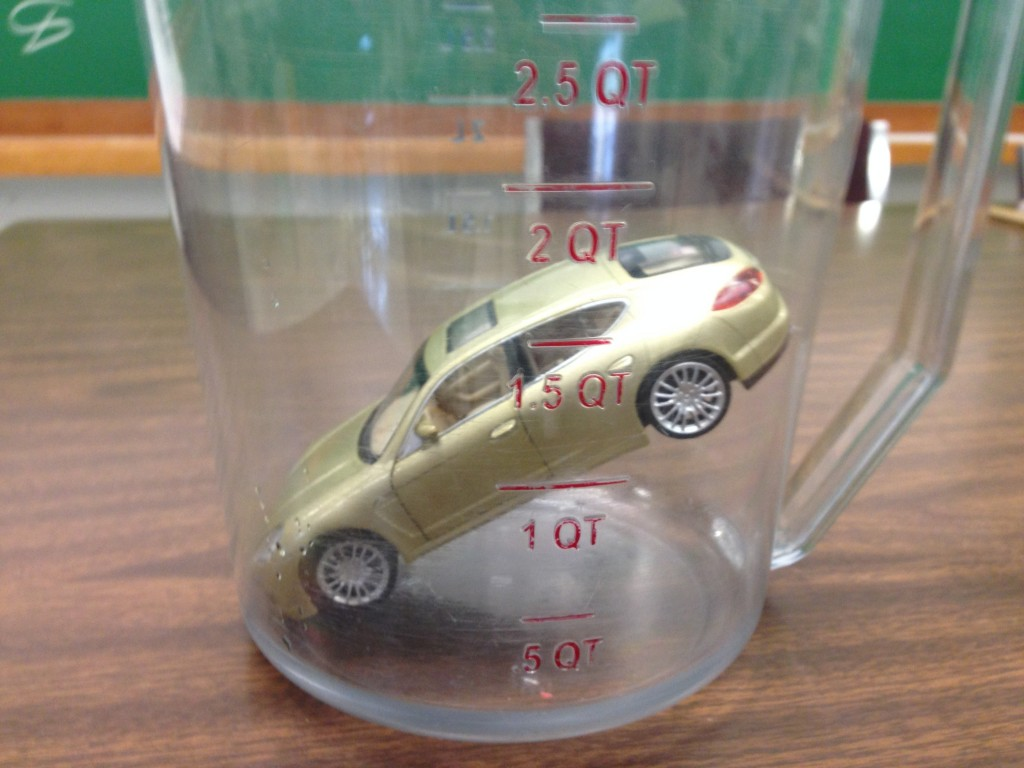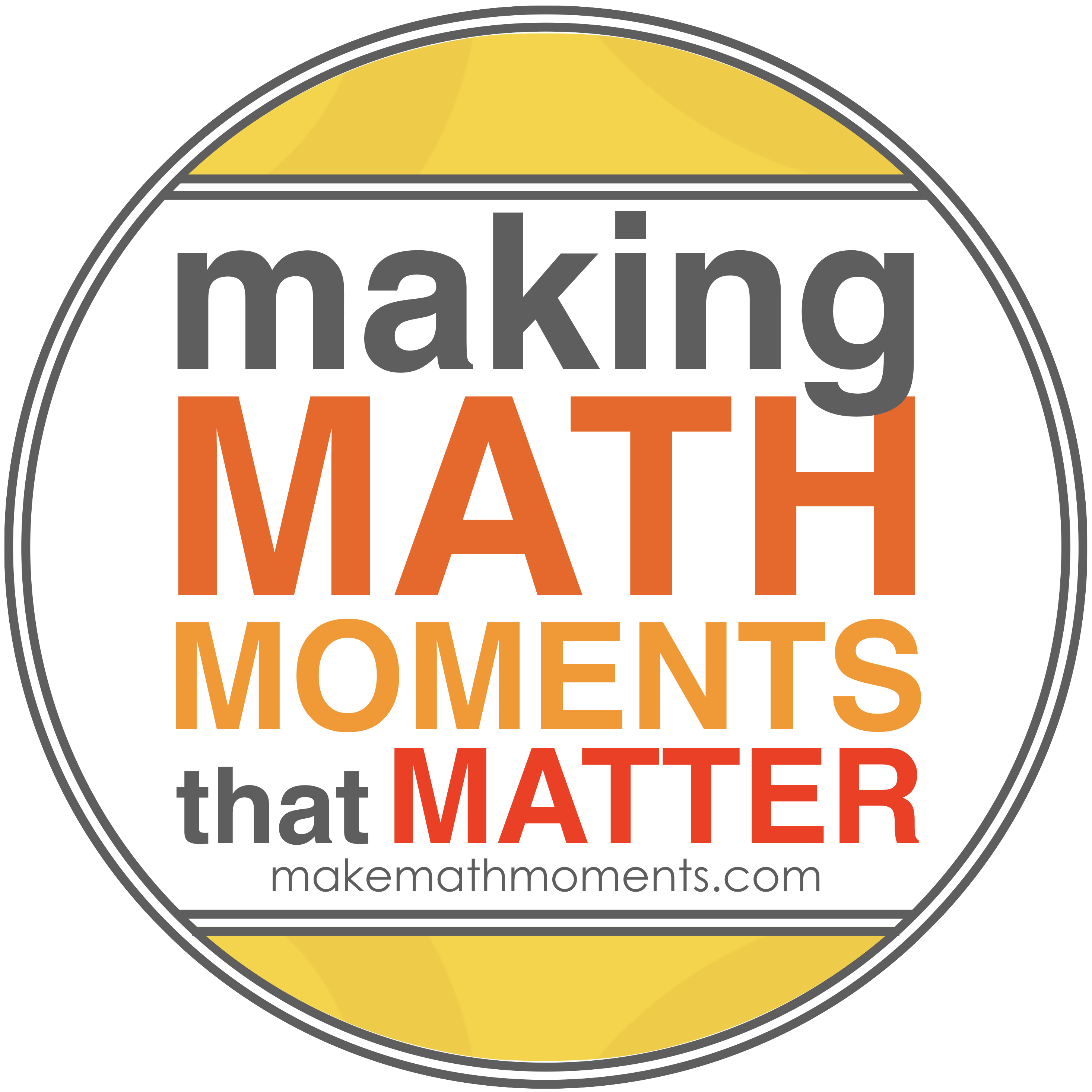# Filling it up!In our grade 9 applied class we are finishing off linear relations and moving into solving equations. I want an activity that is hands-on, engaging, and shows a purpose to solving linear equations.

Here is some thoughts on an activity I want to try. Let me know what you think. Any feedback would be greatly appreciated.

Here it is: Filling it up!!

Show them this picture….Let them wonder, let them ask what that thing in the pitcher is.

ME:

“How many would be too much?”

“How many would be not enough?”

“How many is just right?”

Have them record the guess. “We’ll compare our answer to our guess”

Next,

ME: Let’s find out how many.

Organize them into groups of 3.

ME: What are we going to need?

We’ll need volume of the pitcher, volume of the cup, and volume of the weight.

Have discussion on:

What shape is the cup? …..is it more like a cylinder or a cone? Which is it closest to? What formula for volume will you use? Will you be right?

What shape is the pitcher? What shape is the weight?

Choose 1 member of you group to find the volume of the cup; choose 1 member for volume of the pitcher; choose 1 member to find volume of the weight.

Have the items around the room like stations:Each member will find the volume of their object and bring it back to the group.

Allow the students to work

Here are some scaffolding questions I can use (Please feel free to give me some more)

• What’s changing as you fill up the pitcher?
• What volume is left after the weight?

Here is a possible solution….My idea is this could be great context for introducing solving equations using opposite operations! Use their technique  and show how the volume grows as the cups increase. Use Desmos and relate it to y = mx + b.show them how their strategy is the same as solving 5562 = 1511 + 335x. Boom! Context for solving equations!

My ideas for extensions would be to put objects like….in the pitcher. Count how many cups to fill the pitcher now. Use our equation to solve for the volume of the car. [Corresponding Grade 9 Academic learning goal: Find the y-intercept (initial value) of a linear equation given the slope (rate of change) and a point. ]

What do you think?? Think it would work? I would love some feedback!

## 6 thoughts on “Filling it up!”

1.Kyle Pearce

Really like this one!

My goal next unit in grade 9 applied is to find ways to make the kids “need” to solve the equation to answer a burning question. I think this could do the trick! I wonder if a bit of scaffolding for my 1P’s might be necessary to help them along with the pitcher/cup volume as they will likely have some trouble coming up with a strategy.

When are you intending to do this? Maybe we can do some student sharing?

1.Mr. Orr

I just did it with 1ps today. I asked them what they needed and they came up with the three volumes and went to work! I was very surprised when each group subtracted the weight volume from the pitcher then divided. This seemed natural to them. I’ll keep you posted!

2.great work here, I love the idea of adding an object to change the starting volume and drive the concept of a linear function. Any advice on where to buy pitchers for measuring? Also, were there any issues with the calculations since the pitcher isn’t actually cylindrical? Or were the calculations close enough?

1.Jon Orr

Thanks, I just used a pitcher from our culinary arts class. The idea that the pitcher isn’t perfect was a great discussion….same with the styrofoam cups. I asked which is it closer to a cone or a cylinder? We agreed cylinder. At the end it was ok we were off…we knew we would be!

3.Lee McNeese

Tried this lesson with my students yesterday and today. It went well. There was some discussion back and forth on whether to add the can volume or subtract. After a while I stepped in with the hand circle and marker strategy to help them see how the volume changed. This helped. As you suspected one student multiplied the radius by two instead of squaring. I found the need to measure myself and do the calculations since after actually pouring water into the containers the numbers were far off from the calculations. I discovered the cup measurements were incorrect. Next time I will measure everything myself so I can check student work. I really liked this assignment and so did the students. Thanks.

4.It might be obvious but its always a good idea to label the graph and describe what are X1 and Y1## CONTACT ME